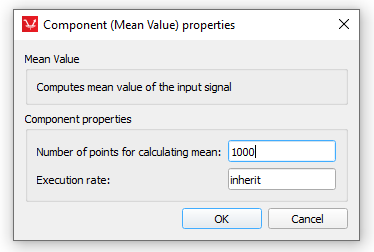# Mean Value

Description of the Mean Value component in Schematic Editor, which outputs the mean value of an input signal.

## Description

The Mean Value component outputs the mean value of a input signal.

For a given input signal of the Mean component x(t), the output signal y(t) of the Mean value component is given by:

where TP is the period of input signal x(t). The period is calculated by multiplying the number of points and the execution rate.

## Ports

• Input (in)
• Input signal
• Supported types: real
• Vector support: no
• Output (out)
• Mean value of the input signal
• Supported types: real
• Vector support: no

## Properties• Number of points
• Enter the number of points for which you want to calculate the mean value.
• Execution rate
• Type in the desired signal processing execution rate. This value must be compatible with other signal processing components of the same circuit: the value must be a multiple of the fastest execution rate in the circuit. There can be up to four different execution rates, but they must all be multiple of the basic simulation timestep. To specify the execution rate, you can use either decimal (e.g. 0.001) or exponential values (e.g. 1e-3) in seconds. Alternatively, you can type in ‘inherit’ in which case the component will be assigned execution rate based on the execution rate of the components it is receiving input from.